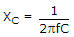# Electronics - Capacitors - Discussion

### Discussion :: Capacitors - General Questions (Q.No.2)

2.

Select the equation below that represents the relationship between charge, capacitance, and voltage for a capacitor.

 [A]. Q = CV [B]. C = QV [C].[D]. V = IR

Explanation:

No answer description available for this question.

 Ravin Birla said: (Nov 12, 2010) How can we justify?

 Guru said: (Mar 3, 2011) Generally the capacitor equ is C=Q/V. Then how?

 Prasanna said: (Jul 6, 2011) Q=CV is the correct answer.

 Vinod said: (Oct 2, 2011) The amount of charge raised or decrease by voltage. It is electrical charge stored element.

 Muhammad Jamal said: (Feb 15, 2012) Capacitors are used to store charge. The amount of charge raised or decrease by voltage. That's why there is a direct relation between charge and voltage. And the equation below best describes the relation. Q=CV.

 Divya Kashyap said: (Feb 7, 2013) Charge stored in a capacitor is directly proportional to the the voltage applied across it. So Q is directly proportional to V. And C is the proportionality constant so the answer is Q=CV.

 Baskaran.R said: (Aug 29, 2013) The charge in the capacitor is raised and reduced by the amount of voltage applied. So the charge is directly proportional to the voltage.

 Mahinder Singh said: (Nov 12, 2013) We donate capacitance charge to Q and donate capacitor voltage to the Cv and the answer is Q=Cv.

 Tanmoypramanik said: (Jul 17, 2014) We donate capacitance charge to Q and donate capacitor voltage to the Cv and the answer is Q=Cv.

 Siva said: (Dec 1, 2016) It should be Q = CV^2.

 Ravisingh said: (Jul 9, 2017) Well said @anmoypramanik.